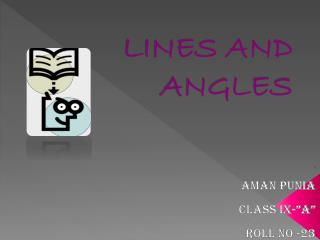DownloadDownload PresentationLINES AND ANGLES

# LINES AND ANGLES

Download Presentation## LINES AND ANGLES

- - - - - - - - - - - - - - - - - - - - - - - - - - - E N D - - - - - - - - - - - - - - - - - - - - - - - - - - -
##### Presentation Transcript

1. LINES AND ANGLES : AMAN PUNIA CLASS IX-”A” ROLL NO -23

2. B A D l C m PARALLEL LINES • Def: line that do not intersect. • Illustration: • Notation:l || mAB|| CD

3. Examples of Parallel Lines • Hardwood Floor • Opposite sides of windows, desks, etc. • Parking slots in parking lot • Parallel Parking

4. Examples of Parallel Lines • LINE A • LINE B • HERE LINE A and LINE B Are PARALLEL

5. m n PERPENDICULAR LINES • Def: Lines that intersect to form a right angle. • Illustration: • Notation:m n • Key Fact: 4 right angles are formed.

6. Ex. of Perpendicular Lines • Window panes : The Window Panes are • PERPENDICULAR to each other

7. Transversal • Def: a line that intersects two lines at different points • Illustration: t

8. Vertical Angles • Two angles that are opposite angles. t • 1=4 • 2 = 3 • 5 = 8 • 6 = 7 1 2 4 3 6 5 7 8

9. Vertical Angles • Find the measures of the missing angles t 125  ? 125  55  ? 55 

10. t 1 2 4 3 6 5 7 8 Supplementary Angles/Linear Pair • Two angles that form a line (sum=180) • 1+2=180 • 2+4=180 • 4+3=180 • 3+1=180 • 5+6=180 • 6+8=180 • 8+7=180 • 7+5=180

11. Supplementary Angles/Linear Pair • Find the measures of the missing angles t ? 108  72  180 - 72 ? 108 

12. 1 2 3 4 5 6 7 8 Corresponding Angles • Two angles that occupy corresponding positions. t • 1= 5 • 2 = 6 • 3 =  7 • 4 = 8 Top Left Top Right Bottom Left Bottom Right Top Left Top Right Bottom Left Bottom Right

13. Corresponding Angles • Find the measures of the missing angles t 145  35  ? 145 

14. Alternate Interior Angles • Two angles that lie between parallel lines on opposite sides of the transversal t • 3 = 6 • 4 = 5 1 2 3 4 5 6 7 8

15. Alternate InteriorAngles • Find the measures of the missing angles t 82  82  98  ?

16. Alternate Exterior Angles • Two angles that lie outside parallel lines on opposite sides of the transversal t • 2 =  7 • 1 =  8 1 2 3 4 5 6 7 8

17. Alternate ExteriorAngles • Find the measures of the missing angles t 120  ? 120  60 

18. Consecutive Interior Angles • Two angles that lie between parallel lines on the same sides of the transversal t • 3 +5 = 180 • 4 +6 = 180 1 2 3 4 5 6 7 8

19. Consecutive InteriorAngles • Find the measures of the missing angles t 180 - 135 135  45  ?

20. THANK YOU  THANK YOU 